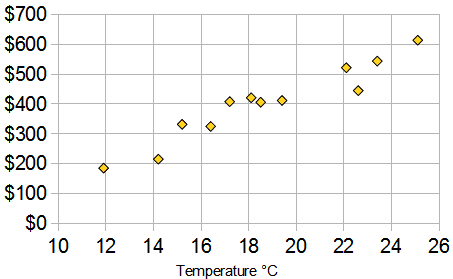Standard

The graphs are about having data for two variables (x, y) . Typically the two types of data are related in some way.

Below is a Scatter Plot with bivariate data. The two things that we are comparing are Temperature and Ice Cream Sales.

Here we have ice cream sales versus the temperature on that day. The two variables are Ice Cream Sales and Temperature.

(If you have only one set of data, such as just Temperature, it is called “Univariate Data, bivariate mean two types of data)In this scatter plot you can see that as tempurate rises so do prices.

https://www.mathsisfun.com/definitions/bivariate-data.html

Click here and  here   and here and here to practice Identifying Scatter Plot Trends and Predicting with Best Fit Lines online and get automatic feedback (it grades it)! 🙂

Writing Linear Equations

Slope Intercept Form:      y = mx + b

The variable m is the slope, it explains the steepness of a line.

The variable b is the y-intercept, this is where the line touches or intersects at the y-axis.

http://www.shmoop.com/video/slope-intercept-form/